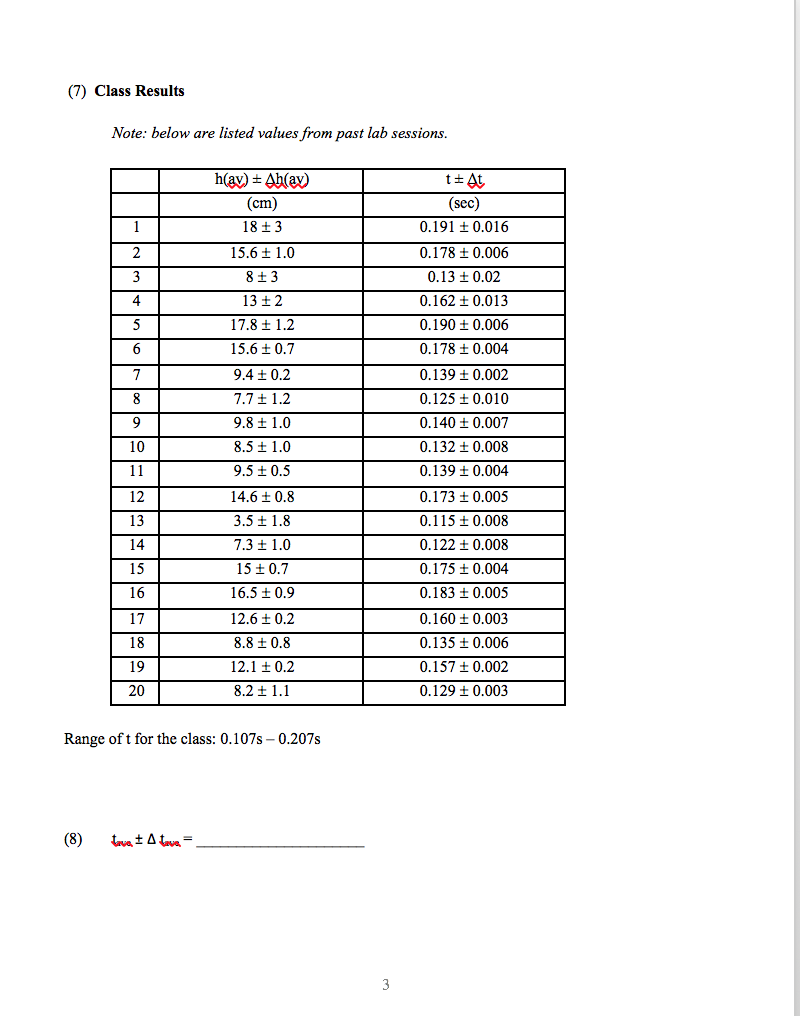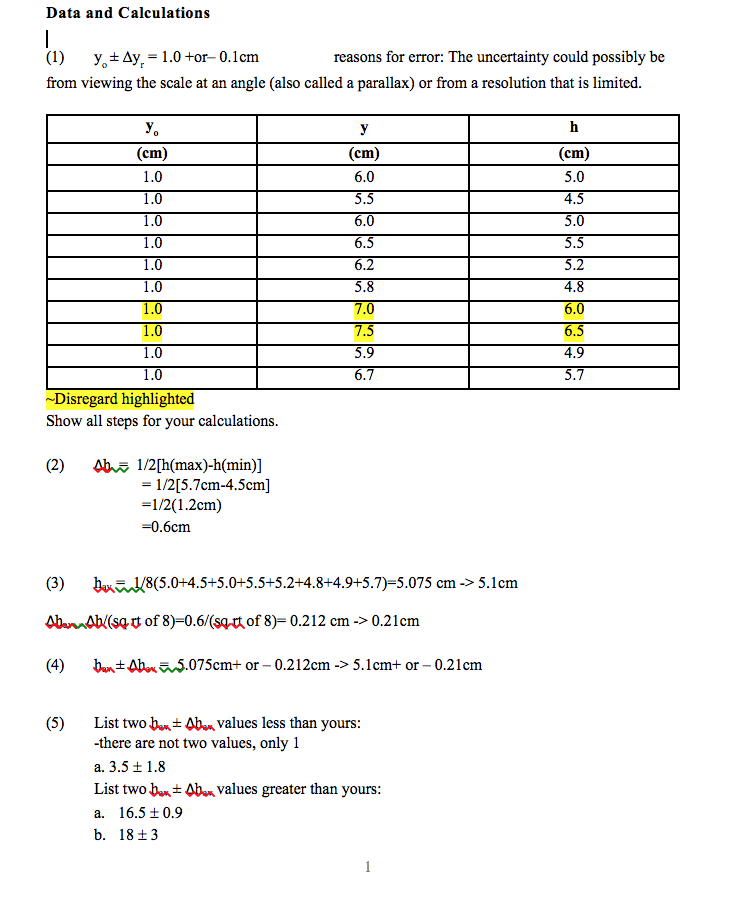# any help appreciated! especially checking with what i already have for sig figs! (2)    Calculate the approximate random error ∆h = (1/2) [h(max) - h(min)], where h(max) and h(min) are the highest and...

any help appreciated! especially checking with what i already have for sig figs!

(2)    Calculate the approximate random error ∆h = (1/2) [h(max) - h(min)], where h(max) and h(min) are the highest and lowest values of h. ∆h refers to the random error in each measurement of h.

(3)    Calculate the average hav = (1/8)[h1 + h2 +…+ h8] and the error in the average ∆hav = ∆h/√8, of the eight trials. ∆hav gives an overall estimate for the random error in the eight repeated measurements of h.

(4)    Write down your value of hav ± ∆hav according to the rules of significant figures.

(7)    What is the range of reaction times from the Class Results table?(8)       Using the measurements listed on the table, calculate the average human reaction time and the error in that average

(7) Class Results Note: below are listed values from past lab sessions (sec) 0.191 t 0.016 0.178 t 0.006 0.13 t 0.02 0.162 t 0.013 0.190 ± 0.006 0.178 t0.004 0.139 ± 0.002 0.125 0.010 0.140 t 0.007 0.132 t 0.008 0.139 t 0.004 0.173 t0.005 0.115 t 0.008 0.122 t 0.008 0.175 t0.004 0.183 ± 0.005 0.160 t 0.003 0.135 t 0.006 0.157 t 0.002 0.129 t0.003 cm 18+3 15.6t 1.0 8+3 13 12 17.8 t1.2 15.6 ± 0.7 9.4 t 0.2 7.7 + 1.2 9.8 1.0 8.5 t 1.0 9.5 10.5 14.6 + 0.8 3.5 + 1.8 7.3 + 1.0 15 t 0.7 16.5 t 0.9 12.6 ± 0.2 8.8 t0.8 12.1 t 0.2 8.2 ± 1.1 4 10 12 13 15 16 17 18 19 20 Range oft for the class: 0.107s - 0.207s
Data and Calculations (I) y,-Ay,-1.0+or0.lcm from viewing the scale at an angle (also called a parallax) or from a resolution that is limited. reasons for error: The uncertainty could possibly be (cm) 5.0 4.5 5.0 5.5 5.2 4.8 cm 1.0 1.0 1.0 1.0 1.0 1.0 cm 6.0 5.5 6.0 6.5 5.8 5.9 6.7 4.9 1.0 5.7 Disregard highlighted Show all steps for your calculations 1/2[5.7cm-4.5cm] 1/2(1.2cm) -0.6cm (3) Abu (4) b8(5.0+4.5+5.0+5.5+5.2+4.8+4.9+5.7)-5.075 cm-> 5.1cm Ab/(sqt of8)-0.6/(sa-rt of 8)-0.212 cm -> 0.21cm h^ Oba%2.075cm+ or _ 0.21 2cm-> 5. 1 cm+ or-0.21 cm (5)List two bAbn values less than yours there are not two values, only 1 a. 3.5 1.8 List two ban-Abn values greater than yours a. 16.5±0.9 b. 18+3

2. dh = 0.5(hmax - hmin) = 0.6 cm
3. hav = dh/sqrt(8) = 0.21 cm
4. hav + dhav = 0.6 +- 0.21 cm
7. range for t = 0.107 - 0.207 s
8. from the given data
0.191   0.0016
0.178   0.006
0.13   0.02
0.162   0.013
0.19   0.006
0.178   0.004
0.139   0.002
0.125   0.01
0.14   0.007
0.132   0.008
0.139   0.004
0.173   0.005
0.115   0.008
0.122   0.008
0.175   0.004
0.183   0.005
0.16   0.003
0.135   0.006
0.157   0.002
0.129   0.003

tav = 0.15265 = (t1 + t2 .. tn)/n
n = 20
tmax = 0.191
tmin = 0.115
dt = 0.5[tmax - tmin] = 0.038
dtav = dt/sqrt(n) = 0.008497

hence reaction time = 0.038 +- 0.00-8497 s